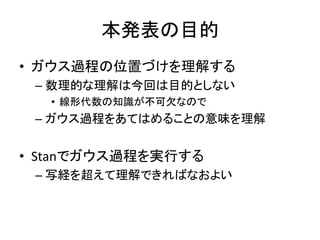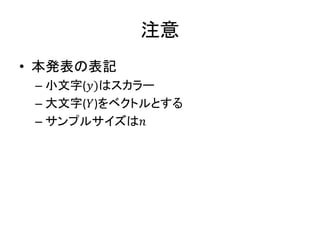Successfully reported this slideshow.

# Stanでガウス過程

13

Share×
1 of 50
1 of 50

# Stanでガウス過程

13

Share

ベイズ塾春の合宿で発表した資料です。

ベイズ塾春の合宿で発表した資料です。

## More Related Content

### Related Books

Free with a 30 day trial from Scribd

See all

### Related Audiobooks

Free with a 30 day trial from Scribd

See all

### Stanでガウス過程

1. 1. Stanでガウス過程 清水裕士 関西学院大学
2. 2. 本発表の目的 • ガウス過程の位置づけを理解する – 数理的な理解は今回は目的としない • 線形代数の知識が不可欠なので – ガウス過程をあてはめることの意味を理解 • Stanでガウス過程を実行する – 写経を超えて理解できればなおよい
3. 3. 注意 • 本発表の表記 – 小文字(𝑦)はスカラー – 大文字(𝑌)をベクトルとする – サンプルサイズは𝑛
4. 4. ガウス過程のモチベーション
5. 5. 一次式 破線は予測区間
6. 6. 2次式で推定
7. 7. 3次式
8. 8. 4次式
9. 9. 何次式がいいだろうか？ • データに合わせすぎる – たとえば4次式 – オーバーフィッティング • モデルが単純すぎる – たとえば2次式 – モデルと予測が乖離
10. 10. オーバーフィッティング • 今回のデータに予測を合わせすぎてしまう – データはサンプリングの変動を必ず含む – たまたま高めだったり低めだったりする – そういう「たまたま」を「真のメカニズム」と判断してし まい、予測してしまうと、外れる • パラメータの複雑さについてのジレンマ – WAICなどの汎化性能を評価する指標も使える – データから直接いい感じに推定したい – →ガウス過程回帰
11. 11. ガウス過程を一言でいうと • データにうまくフィットする関数を推定する – 関数系そのものを推定する – 𝑦𝑖 = 𝑓(𝑥𝑖) • 𝑖 ∈ 1 … 𝑛 • 普通の線形回帰 – 𝑦𝑖 = 𝑤0 + 𝑤1 𝑥𝑖 + 𝑤2 𝑥𝑖 2 … 𝑤 𝑚 𝑥𝑖 𝑚 – どういう関数形にすればいいのかわかんない – それ自体を推定するのがガウス過程
12. 12. ざっくりいえば平滑化手法 • ヒストグラムを平滑化 – カーネル密度推定 • 予測モデルを平滑化 – カーネル回帰 • これらを確率モデルで表現すると・・ – ガウス過程という方法が浮かび上がってくる
13. 13. 古典的平滑化の例 • カーネル密度推定
14. 14. 古典的ノンパラ手法の限界 • 確率モデルの長所を生かせない – モデル評価がしにくい – ハイパーパラメータの最適化が困難
15. 15. ガウス過程を使うと・・ • 自動的に平滑化＋予測区間の推定
16. 16. 平滑化も確率モデルで！ • まずはカーネル法の導入 – モデルの見方をちょいと変えれば、パラメータを 消去して、直接データを予測することが可能 – カーネルトリック！ • カーネルトリックの話はちょっと難しい・・ – 理解するにはちょっと数学的な素養が必要 – カーネルトリックがわからなくてもガウス過程は使 える
17. 17. 確率モデルということは・・・ • Yes,Bayesian! – ベイズ推定が可能 – ノンパラメトリックベイズとは、要は確率過程を用いた ベイズ推定のこと • ガウス過程 • ディリクレ過程 • ガウス過程を使うと・・・ – ノンパラモデルをベイズ推定可能になる – ハイパーパラメータの自動学習もできる
18. 18. ガウス過程は機械学習の奥義 • ほとんどの機械学習はガウス過程で表現可能 – サポートベクターマシーン – ディープラーニング • 中間層のニューロン無限のDNN – カーネル回帰分析 • ガウス過程回帰 – カーネル主成分分析 • ガウス過程潜在変数モデル（GPLVM） • 統計のプロからのコメント – ガウス過程はなんでも上手くいくからおもんない
19. 19. ガウス過程回帰分析
20. 20. 線形回帰とカーネル回帰 • 普通の線形回帰 – データ𝑌に対して𝑋で線形モデルをあてはめる • 𝑋は𝑥の𝑚次元多項式 – パラメータ𝑤を求める(切片、回帰係数） – 𝑦𝑖 = 𝑤0 + 𝑤1 𝑥𝑖 + 𝑤 𝑤 𝑥2 + ⋯ + 𝑤 𝑚 𝑥 𝑚 • 𝑌 = 𝑋𝑊
21. 21. 基底関数 • 基底関数を使った表現 – 任意の𝑥の基底関数を𝜙(𝑥)とする • 基底関数・・・𝑥 𝑎 とかsin(𝑥)とかみたいに𝑥から一意に決まる 変換関数 • ここでは上と同じように多項式（べき関数）だけを考えてOK • 基底関数を使って書き直す – 𝑦𝑖 = 𝑤0 + 𝑤1 𝜙1(𝑥) + 𝑤2 𝜙2(𝑥) + ⋯ + 𝑤 𝑚 𝜙 𝑚(𝑥) – 𝑌 = ΦW – パラメータ𝑤と𝑥、基底関数𝜙から予測値ො𝑦を推定する
22. 22. 確率モデルを考える • 𝑤が正規分布に従うと仮定する – 𝑤~𝑁𝑜𝑟𝑚𝑎𝑙 0, 𝜆𝐼 𝑚 • 𝐼 𝑚は𝑚次元単位行列 • 𝜆はスカラー • これはリッジ回帰と同じ仮定 • 𝑤の確率分布から、𝑌の確率分布を導く – 𝑌は線形結合による関数なので、𝑌も正規分布にな ることが想像できる
23. 23. Yの平均と分散を計算する • 𝑌の平均と共分散を計算する – 𝜇 𝑌 = 𝐸 𝑌 = 𝐸 Φ𝑊 𝑇 = Φ𝐸 𝑊 𝑇 = 𝟎 – 𝑛次元の0ベクトル • 𝑌の共分散行列 – Σ 𝑌 = 𝐸 𝑌𝑌 𝑇 − 𝐸 𝑌 𝐸 𝑌 𝑇 = 𝐸[Φ𝑊 Φ𝑊 𝑇] = Φ𝐸[𝑊𝑊 𝑇]Φ 𝑇 = 𝜆Φ𝐸 𝐼 𝑚 𝐼 𝑚 𝑇 Φ 𝑇 = 𝜆ΦΦ 𝑇 • 変数×変数ではなく、サンプル×サンプルの共分散行列を考える • 𝑌の確率モデル – 𝑌 ~ 𝑀𝑢𝑙𝑡𝑖𝑁𝑜𝑟𝑚𝑎𝑙(𝟎, 𝜆ΦΦ 𝑇 ) – 𝑛変量正規分布
24. 24. カーネルトリック • 共分散行列𝜆ΦΦ 𝑇 に注目 – 𝑛 × 𝑛の正方行列で、正定値なものであればなんでも いい – つまり、基底関数行列Φがなんであっても、最終的に はなんらかの共分散行列を指定するだけで、𝑦の確 率モデルが構成できる • カーネル関数にすべてを還元 – 𝜅 𝑥, 𝑥′ = 𝜆ΦΦ 𝑇 – 関数系Φを具体的に指定せずとも、任意のカーネル 関数を使って共分散行列𝜅 𝑥, 𝑥′ を作ればよい
25. 25. カーネルトリック • 内積をとることのメリット – ΦΦ′つまり𝑥についての多項式の内積をとると、たか だか𝑛次元の多変量正規分布を考えるだけでよい • 無限次元の多項式（一般には基底）関数が、有 限次元のカーネル関数で表現できる – カーネルトリック！ – 𝑚は無限次元でも、データの次元を超える関数形を 考える必要がない、という点がポイント
26. 26. ガウス過程の確率モデル • 𝑌~𝑀𝑢𝑙𝑡𝑖𝑁𝑜𝑟𝑚𝑎𝑙 𝟎, 𝜅(𝑥, 𝑥′ ) – サンプルサイズが𝑛の場合、𝑛変量正規分布を考 える – 𝜅(𝑥, 𝑥′)は𝑛×𝑛の共分散行列を構成する関数 • カーネル関数と呼ぶ • この共分散行列をどのように決めればいい？ – ガウス過程はカーネル関数をどうするかがすべ てといって過言ではない
27. 27. カーネル関数 • ここからが重要！ – ガウス過程のほぼすべてがここに詰まっている • データポイント間の相関を表現する関数 – 予測変数𝑥とハイパーパラメータを使って、データ ポイント間の近さを表現するような行列を作る
28. 28. 例：とある時系列データ • 20個のデータ – 20次元変量正規分布を考える – 任意の２点のデータポイントと類似性を表す 𝑥の距離が近いほど𝑦の相関が高くなるようにすれば なめらかな予測が可能
29. 29. ガウスカーネル • よく使われるのがガウスカーネル – 𝜅 𝑥, 𝑥′ = 𝜃1 exp − 𝑥−𝑥′ 2 2𝜃2 • ガウスカーネルとガウス過程は別物なので注意！ • exp − 𝑥−𝑥′ 2 2 の部分が正規分布の密度関数のカー ネルと一致している – RBFやexponential quadric kernelなどと呼ばれる
30. 30. ガウスカーネルの例 x <- seq(1,5) n <- length(x) theta <- 10 kappa <- array(dim=c(n,n)) for(i in 1:n){ for(j in 1:n){ kappa[i,j] <- exp(-(x[i]-x[j])^2/(2*theta)) } } kappa 近い点ほど 相関が大き い
31. 31. 距離が離れると相関が減る
32. 32. ガウスカーネルのハイパーパラメータ • 𝜅 𝑥, 𝑥′ = 𝜃1 exp − 𝑥−𝑥′ 2 2𝜃2 – 𝜃1ガウスカーネル自体がどれくらいカーネル関数全 体に寄与しているかを表している – 𝜃2ガウスカーネルのハイパーパラメータで、距離に応 じてどれくらい相関が小さくなるかの程度 • 𝜃2が大きいほど、なめらかな予測になる – ただし、これらはすべてMCMCで推定可能 • なんらかの方法で事前に決めてもいい
33. 33. ガウスカーネルで推定 • なめらかに予測を行うことができる
34. 34. それ以外にもカーネル関数はある • 線形カーネル – 𝜅 𝑥, 𝑥′ = 𝜃𝑥𝑥′ x <- seq(1,5) n <- length(x) kappa <- x%*%t(x) kappa 任意の2点の相関が1 xの分散の応じて分散は 大きくなる 任意のべき乗をいれれば、 多項式回帰と同じにできる
35. 35. それ以外にもカーネル関数はある • フィッシャーカーネル • シグモイドカーネル • ニューラルネットカーネル – これはよく知らん！
36. 36. カーネルを組み合わせる • よく使われる組み合わせ – ガウス＋線形＋ノイズ – 𝜅 𝑥, 𝑥′ = 𝜃1 exp − 𝑥−𝑥′ 2 2𝜃2 + 𝜃3 𝑥𝑥 𝑇 + 𝑑𝑖𝑎𝑔(𝜃4) – これをStanに書けばOK！
37. 37. ガウス＋線形の推定 • ガウスカーネルだけよりも線形的予測が入る
38. 38. カーネル部分のStanコード 𝜅 𝑥, 𝑥′ = 𝜃1 exp − 𝑥 − 𝑥′ 2 2𝜃2 + 𝜃3 𝑥𝑥 𝑇 + 𝑑𝑖𝑎𝑔(𝜃4) 今回はガウスカーネル＋ノイズで推定
39. 39. 𝑦1と𝑥1のプロット • 𝑥1は1~20の20個 – 𝑦1はそれに対応する目的変数 – 𝑥が21~30のො𝑦を推定したい
40. 40. まずは𝑥2を作る • N2は任意の数 – 推定したい点の数 – 𝑥2は1~30の間を59分割した点 N1 <- nrow(dat) x1 <- dat\$x y1 <- dat\$y N2 <- 59 x2 <- seq(1,30,length.out = N2) data.gp <- list(N1 = N1, N2 = N2, x1 = x1, x2 = x2, y1 = y1)
41. 41. x2 • この点についてො𝑦を推定する – 𝑦1に対応する𝑥1と被っていても問題ない – むしろ、𝑦1の予測値ො𝑦を知りたいこともあるので、 被らせるほうがいいかも – イメージとしては、既知の𝑦を使ってカーネルのパ ラメータを推定し、ො𝑦をそこから推定する感じ
42. 42. コードの解説 • N1 – サンプルサイズ – 𝑥1と𝑦1は実際にデータとしてとったもの • N2 – 推定したいො𝑦のサイズ – X2は推定したいො𝑦に対応する𝑥
43. 43. コードの解説 • Nはデータ＋推定したい点の総数 – すでにあるデータを使って ො𝑦 を推定するためには、 ベクトルを合成しておく必要がある
44. 44. コードの解説 • theta – カーネル関数のハイパーパラメータ • 今回は3つ • 𝑦2 – 推定したいො𝑦
45. 45. コードの解説 • ガウス過程の確率モデル – シンプルに平均0の多変量正規分布 xの分散を入れることでthetaが大きくな りすぎるのを防いでいる なくても推定は可能
46. 46. 最終的なRコード dat <- read.csv("gp_test.csv") N1 <- nrow(dat) x1 <- dat\$x y1 <- dat\$y N2 <- 59 x2 <- seq(1,30,length.out = N2) data.gp <- list(N1 = N1, N2 = N2, x1 = x1, x2 = x2, y1 = y1) model.gp <- stan_model("GP_reg.stan") fit.gp <- sampling(model.gp, data = data.gp, iter = 5000, chains = 4, cores = 4)
47. 47. なめらかーに予測 カエルの卵みた いとか言わない
48. 48. プロットのためのコード y2.med <- apply(ext.fit\$y2, 2, median) y2.low <- apply(ext.fit\$y2, 2, quantile, probs = 0.05) y2.high <- apply(ext.fit\$y2, 2, quantile, probs = 0.95) d.est <- data.frame(x=x2, y=y2.med, ymax=y2.high, ymin=y2.low) d.obs <- data.frame(x=x1, y=y1, ymax=rep(0,N1), ymin=rep(0,N1)) p <- ggplot(d.est, aes(x = x, y = y, ymax=ymax, ymin=ymin)) p <- p + xlab("x") + ylab("y") p <- p + geom_ribbon(alpha = 1/3) p <- p + geom_line(aes(y = y), color = "blue") p <- p + geom_point(data = d.obs) p
49. 49. ハイパーパラメータ • いい感じに推定してくれる – フルベイズのいい点
50. 50. まとめ • ガウス過程 – 回帰の関数を明示的に決めなくても、いい感じの予 測を自動でしてくれるテクニック – カーネル関数はいろいろあるので、うまくいくものを組 み合わせてみよう • 回帰以外も使える – 密度関数や潜在変数モデル、クラスタリングなど、い ろんな分野にガウス過程は使える – （若干遅いが）Stanで簡単に実装可能